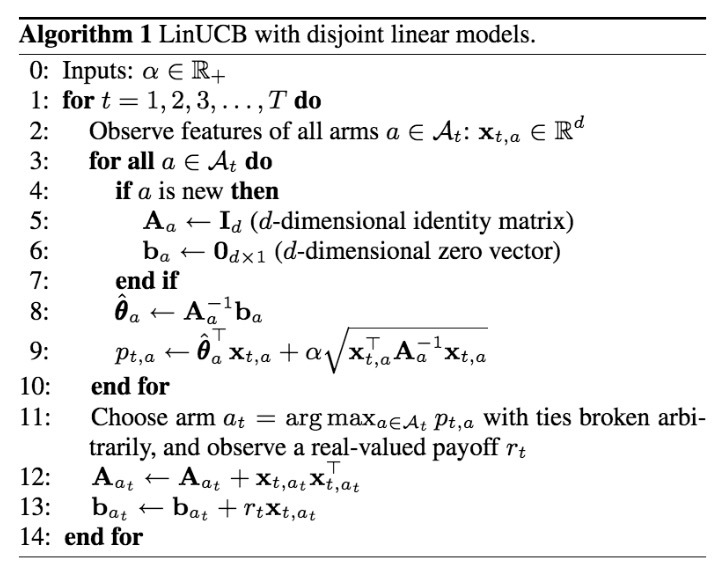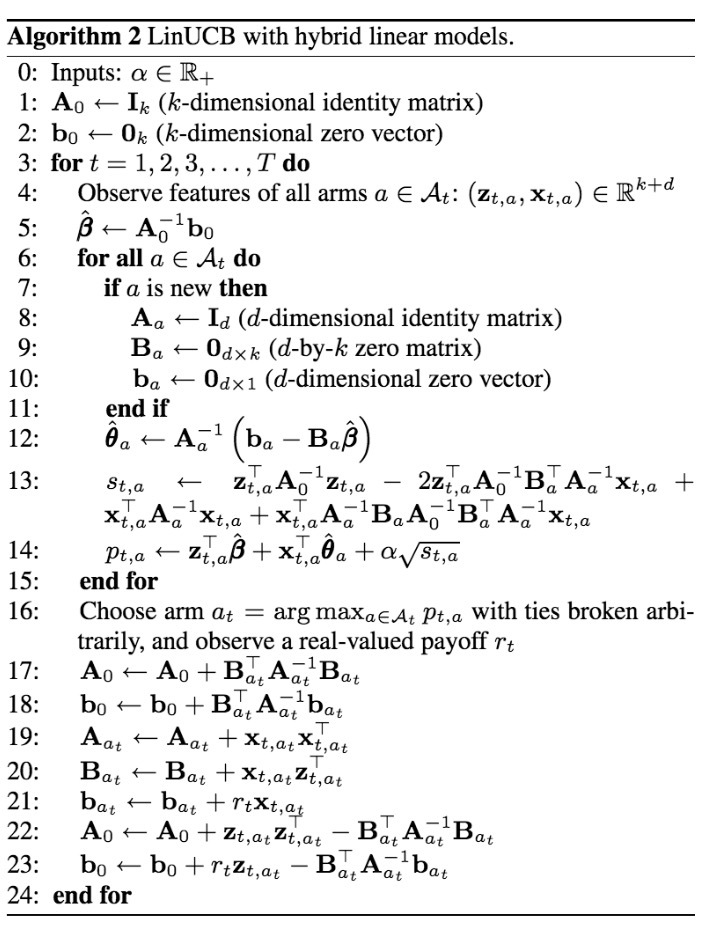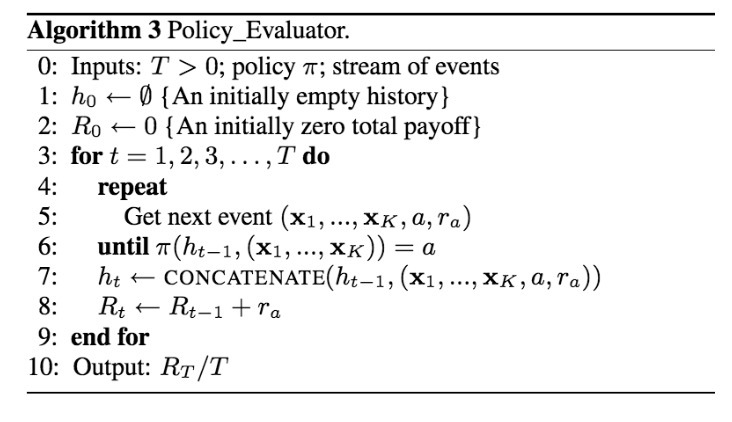LinUCB介绍

August 12, 2016

yahoo在2010年在《A contextual-bandit approach to personalized news article recommendation》中介绍了contextual bandit算法。

2.公式&相关工作

2.1 Multi-armed Bandit公式

• 1.该算法会观察当前用户$u_t$和一个关于arms或actions的$A_t$集合，以及对于arm $a \in A_t$的它们的特征向量(feature vectors) $x_{t,a}$。 vector $x_{t,a}$会同时总结用户$u_t$和arm a的信息，被称为context
• 2.基于在之前实验中的已观察收益(observed payoffs)，算法A会选择一个arm $a_t \in A_t$，并得到新的收益(payoffs): $r_{t,a_t}$，它的期望取决于user $u_t$和arm $a_t$两者
• 3.算法接着使用新的observation $(x_{t,a_t}, a_t, r_{t,a_t})$提升它的arm-selection策略。这里需要重点强调的是：对于未选中的arms $a \neq a_t$，此处没有观察到feedback（也就是：payoff $r_{t,a}$）

$R_A(T) \equiv E[\sum\limits_{t=1}^T r_{t,a_t^*}] - E[\sum\limits_{t=1}^T r_{t,a_t}]$

…(1)

• (i) arm set $A_t$保持不变，对于所有t都包含K个arms
• (ii) user $u_t$（或者相等的，context $x_{t,1}, \cdots, x_{t,K}$）对于所有t都相同

2.2 已存在的Bandit算法

bandit problems的基本挑战是，需要对exploration和exploitation做平衡。为了最小化等式(1)中的regret（越小表示两者越接近），一个算法A会利用（exploits）它的过往经验来选择看起来最好的arm。另一方面，看起来最优的arm可能在实际上是次优的，因为在算法A的知识(knowledge)中是不精准的（imprecision）。为了避免这种不希望的情况，算法A必须通过实际选择看起来次优的arms来进行explore，以便收集关于它们的更多信息（在bandit过程中的step 3在之前的章节已定义）。Exploration可以增加short-term regret，因为会选到一些次优的arms。然而，获得关于arms的平均payoffs信息（例如：exploration）可以重新定义(refine)算法A的arms payoffs，从而减小long-term regret。通常，即不会存在一个纯粹的exploring，也不会存在一个纯粹的exploiting算法，需要对两者做平衡。

context-free K-armed bandit问题已经被统计学家研究过许多。一种最简单和最直接的算法是ε-greedy。在每个实验t中，该算法会首先估计每个arm a的平均payoff $\hat{\mu}_{t,a}$。接着使用概率$1 - e$来选择greedy arm（例如：具有最高payoff估计的arm）；使用概率e来选择一个random arm。在极限上，每个arm会尝试无限次，以便payoff估计$\hat{\mu_{t,a}}$会收敛到具有概率为1的真值(true value)$\mu_a$。另外，通过对e进行适当的衰减(decaying)，每一step的regret $R_A(T)/T$会收敛到0, 概率为1.

3.算法

3.1 不相交(disjoint)线性模型的LinUCB

$E[r_{t,a} | X_{t,a}] = X_{t,a}^T \theta_a^*$

…(2)

• $D_a$是在实验t上的一个m x d维的设计矩阵(design matrix)，它的行对应于m个训练输入（例如：对于文章a，之前已经观察到的m个contexts）
• $c_a \in R^m$是相应的响应向量(corresponding response vector) （例如：相应的m个 点击/未点击 user feedback） (注：paper写的是$b_a$而非$c_a$，应该是笔误)

$\hat{\theta}_a = (D_a^T D_a + I_d)^{-1} D_a^T c_a$

…(3)

$| x_{t,a}^T \hat{\theta}_a - E[r_{t,a} | x_{t,a}] | \leq \alpha \sqrt{x_{t,a}^T (D_a^T D_a + I_d)^{-1} x_{t,a}}$

…(4)

$a_t \equiv argmax_{a \in A_t} (x_{t,a}^T \hat{\theta}_a + \alpha \sqrt{x_{t,a}^T A_a^{-1} x_{t-a}})$

…(5)• 1.它的计算复杂度对于arms的数量来说是线性的，对于特征数目最多是三次方。为了减少计算量，我们可以在每一step中更新$A_{a_t}$，周期性计算和缓存$Q_a \equiv A_a^{-1}$，而非实时。
• 2.该算法对于一个动态的arm set来说工作良好，仍能高效运行，只要$A_t$的size不能太大。该case在许多应用中是true的。例如，在新闻文章推荐中，编辑会从一个池子中添加/移除文章，池子的size本质上是个常数。
• 3.尽管不会该paper的重点，我们仍会采用的分析来展示：如果arm set $A_t$是确定的，包含了K个arms，接着置信区间（比如：等式(4)的右手边）会随着越来越多的数据快速减小，接着证明$\hat{O}(\sqrt{KdT})$的强regret bound，匹配满足等式(2)的bandits的state-of-art结果。这些理论结果预示着算法的基础牢固以及高效性。

3.2 Hybrid线性模型的LinUCB

$E[r_{t,a} | x_{t,a}] = z_{t,a}^T \beta^* + x_{t,a}^T \theta_a^*$

…(6)

• $z_{t,a} \in R^k$是当前user/article组合的特征
• $\beta^*$是一个未知的系数向量(coefficient vector)，它对所有arms是共享的• 1.由于算法中使用的构建块（$A_0, b_0, A_a, B_a, b_a$）具有固定的维度，可以进行增量更新，该算法计算十分高效。
• 2.另外，与arms相关联的质量（quatities）在$A_t$中并不存在，因而在计算量上不再相关。
• 3.最后，我们也周期性地计算和缓存了逆($A_0^{-1}$和$A_a^{-1}$)，而非在每个实验尾部来将每个实验的计算复杂度$O(d^2 + k^2)$。

4.评估技术• 如果policy $\pi$选择了与logging policy所选arm的相同的arm a，那么：event仍被添加到历史中，total payoff $R_t$会被更新。
• 如果policy $\pi$选择了与logging policy所选arm所不同的arm，那么：event会被完全忽略，该算法会处理下一event，在该state上无需任何变化。

$\underset{policy\_evalutar(\pi,S)}{Pr} (h_T) = Pr_{\pi, D} (h_T)$

$\underset{policy\_evaluator(\pi,S)}{Pr} (h_{t-1}) = Pr_{\pi,D}(h_{t-1})$

$\underset{Policy\_evaluator(\pi,S)}{Pr} ((x_{t,1}, \cdots, x_{t,K}, a, r_{t,a}) | h_{t-1}) = Pr_D(x_{t,1}, \cdots, x_{t,K}, r_{t,a}) Pr_{\pi(h_{t-1})} (a | x_{t,1}, \cdots, x_{t,K})$

5.实验

5.1 Yahoo! Today模块Today模块是在Yahoo! Front Page（流量最大）的最显著位置的panel，详见图1. 在Today Module上缺省的”Featured” tab会对高质量文章（主要新闻）的1/4进行高亮（highlight）, 而4篇文章通过一个小时级别更新的由人工编辑的文章池中进行选择。如图1所示，在底部存在4篇文章，索引为F1-F4. 每篇文章由一张小图和一个标题进行表示。其中之一会在story位置进行着重展示，它由一个大图、一个标题、一个简介、以及相关链接进行着重(featured)。缺省的，在F1的文章会在story位置强调。一个用户可以点击在story位置上的highlightd文章，如果她对文章感兴趣会读取更多的详情。event被看成是一次story click。为了吸引访问者的注意力，我们想根据个人的兴趣对提供的文章进行排序，对于每个visitor在story位置上highlight最有吸引力的文章。

5.2 实验设置

5.2.1 数据收集

• (i) 提供给用户随机选中的文章
• (ii) user/article信息
• (iii) 在story位置处用户是否对该文章有点击

5.2.2 特征构建

• (i) 人口属性信息(demographic information)：性别（2个分类）、年龄（离散化成10个分段）
• (ii)地理特征（geographic features）：包含世界范围和美国的大约200个大都市；
• (iii)行为类别(behavioral categories)：大约1000个二分类别（binary categories），它们总结了用户在Yahoo!内的消费历史。

• (i) URL类别：数十个分类，从文章资源的URL中推断得到
• (ii) editor类别：数十个主题，由人工编辑打标签总结得到

• 我们首先通过原始的user/article features，来使用LR来拟合一个关于点击率（click probability）的bilinear model，以便$\phi_u^T W \phi_a$来近似用户u点击文章a的概率，其中$\phi_u$和$\phi_a$是相应的feature vectors，W是由LR最优化得到的权重矩阵。
• 通过计算$\psi_u = \phi_u^T W$，原始的user features接着被投影到一个induced space上。这里，用于user u，在$\psi_u$的第i个元素可以被解释成：用户喜欢文章的第i个类别的度（degree）。在induced的$\psi_u$ space中使用K-means算法将用户聚类成5个clusters。
• 最终的user feature是一个6向量（six-vector）：5个条目对应于在这5个clusters中的成员（使用一个Gaussian kernel计算，接着归一化以便他们总和一致），第6个是一个常数特征1.

5.3 算法比较

I.不使用特征的算法

• random: random policy总是会以等概率的方式从池子中选中候选文章之一。该算法无需参数，不会一直学习
• ε-greedy：在第2.2节所述，它会估计每篇文章的CTR；接着，它会选择具有概率e的一篇随机文章，接着选择具有概率1-e的最高CTR估计的文章。该policy只有一个参数e。
• ucb: 如2.2节所述，policy会估计每篇文章的CTR，也会估计它的置信区间，总是会选择具有最高UCB的文章。特别的，根据UCB1，我们会通过$c_{t,a} = \frac{a}{\sqrt{n_{t,a}}}$来计算一篇文章a的置信区间，其中$n_{t,a}$是在实验t之前a被选中的次数，$\alpha>0$是一个参数
• omniscient(无所不知的): 这样的一个policy会从后见之明（from hindsight）达到最好的经验型的context-free CTR。它首先会从日志事件(logged events)中计算每篇文章的经验CTR，当使用相同的logged events评估时，接着总是选择具有最高经验CTR的文章。该算法无需参数，不需要学习。

II.热启动（warm start）算法

• ε-greedy(warm):
• ucb(warm):

III.在线学习user-specific CTR的算法

参考

Updated on

STAR算法介绍

PLE介绍

Published on March 04, 2021

DTS介绍

Published on January 02, 2021